## Chemical Reaction with Autocatalytic Step

exd6
Variables and parameters:

yi(t): concentrations of chemicals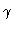: flow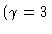): velocity coefficient of autocatalytic step (bifurcation parameter)

Model equations: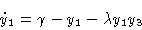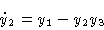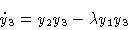Hopf Bifurcation at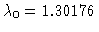with initial period T0=6.03555

Figure 1
Stable solutions,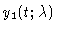for; the time is normalized to unity. Stationary and periodic orbits meet in a Hopf bifurcation.

Figure 2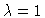, projection to the (y1,y2) plane. Initial values: y1=2, y2=y3=1. Dynamics of a stable focus.

Figure 3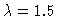, projection to the (y1,y2) plane. Initial values: y1=2, y2=y3=1. Dynamics of a stable limit cycle.

Figure 4
Bifurcation diagram y1(0) versus; Hopf bifurcation at.

This Example
Figure 1
Figure 2
Figure 3
Figure 4